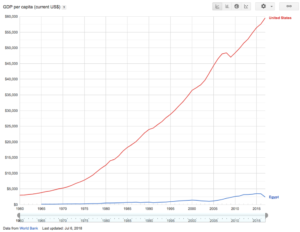How to Price Work in Other Countries

I occasionally use Upwork (formerly eLance) to hire contract workers in other countries to work on some projects. Considering that I live in the San Francisco Bay Area, which happens to be one of the most expensive places to live, I have to constantly remind myself to price my projects according to the economy and cost-of-living where I hire workers overseas. Here’s an example cost analysis.

US Cost
Let’s say that, based on the type of work you need done, a fair US wage would be \$10 per hour. Let’s also say that you expect the work should be done in no more than 17.5 hours. Therefore, the total project cost in the US would be \$175.

Target Country
Let’s say you hire someone in Egypt to do the work. You’ll need a way to fairly and reasonably convert wages in the US to wages in Egypt. One way to do this is by comparing each countries GPD per capita, which is an estimate of the average salary in a particular country.

GDP Per Capita
According to the World Bank, as of July 6, 2018, the GDP per capitas of the US and Egypt are:

• 2018 US GDP per capita = ~ \$60,000
• 2018 Egypt GDP per capita = ~ \$3,000In other words, the average annual salary in the US and Egypt are \$60k and \$3K, respectively.

Cost Ratio
With the GDP per capita data, we can calculate a cost ratio of 3,000 / 60,000 = 1/20 (0.05). In other words, wages in Egypt are 1/20th what they are in the US.

Final Cost
To calculate the cost to hire a worker in Egypt to do the same work in the US, we multiply the US cost by the cost ratio:

\$175 x 1/20 = \$8.75.

Now, \$8.75 may sound like a very small amount of money in the US, and it happens to be less than the US minimum hourly wage, but, since we’re dealing with a very different economy, \$8.75, when converted to local Egyptian currency (Egyptian Pounds), should be a decent amount of money for locals. Nevertheless, to account for possible errors, you can add 25% which gives you

\$8.75 x 1.25 = ~ \$11.

Resources

Here is an online calculator that you can also used to compare salaries.

https://teleport.org/salaries/san-francisco-bay-area/cairo/WEB-DEVELOPER/60000/

According to the link above, a \$60K a year web developer job in San Francisco would be equivalent to a \$3,800 salary for the same job in Egypt after comparing the cost of living between the two locations. Using this result, the cost ratio would be \$3800 / \$60000 = 0.063.

As you can see, this result almost matches the GDP per capita ratio between the two countries calculated earlier (0.05).